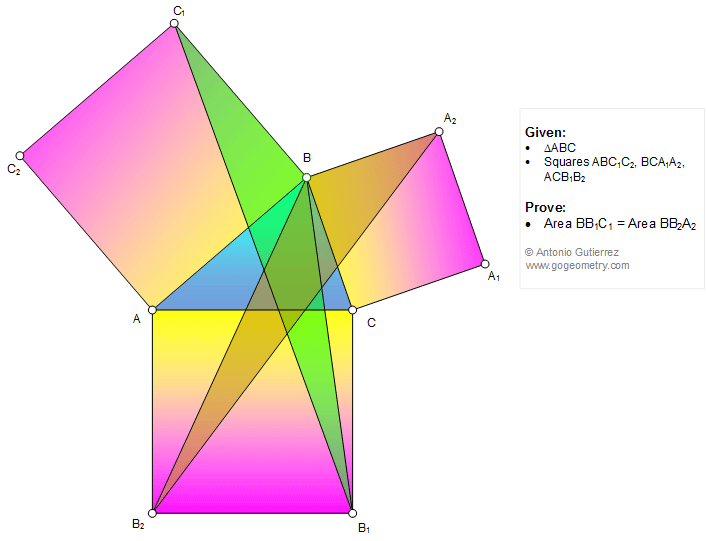# Geometry Problem 1160: Triangle, Three Squares, Area, Equivalent Triangles. Level: School, College, Mathematics Education

< PREVIOUS PROBLEM  |  NEXT PROBLEM >

 The figure below shows a triangle ABC with the squares ABC1C2, BCA1A2, and ACB1B2. Prove that the areas of triangles BB1C1 and BB2A2 are equal (equivalent triangles).Home | Search | Geometry | Problems | All Problems | Open Problems | Visual Index | 10 Problems | Problems Art Gallery | Art | 1151-1160 | Triangles | Square | Triangle with squares | Area of a Triangle | Last updated: Feb 26 2018